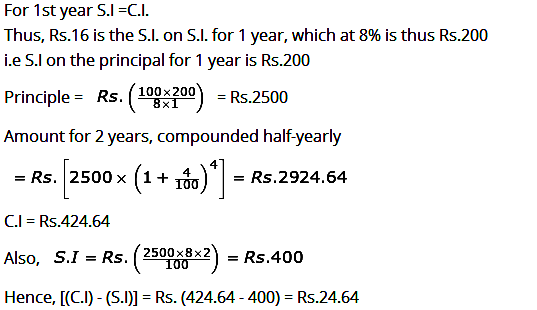Compound Interest Problems Practice Set - 1

Compound Interest Problems Practice Set - 1
1. Ravi Shankar takes money from the employees’ cooperative society at lower rate of interest and saves in a scheme, which gives him a compound interest of 20%, compounded annually. Find the least number of complete years after which his sum will be more than double.
A) 4 years
B) 2 years
C) 6 years
D) 8 years
E) 12 years
2. Equal sums of money are deposited in two different banks by M/s Enterprises, one at compound interest (compounded annually) and the other at simple interest, both at 5% per annum. If after two years, the difference in the amounts comes to Rs. 200, what are the amounts deposited with each bank?
A) Rs.72000
B) Rs.76000
C) Rs.78000
D) Rs.80000
E) Rs.82000
3. Bharat took a loan of Rs. 20000 to purchase one LCD TV set from a finance company. He promised to make the payment after three years. The company charges compound interest at the rate of 10% per annum for the same. But, suddenly the company announces the rate of interest as 15% per annum for the last one year of the loan period. What extra amount does Rohit have to pay due to this announcement of the new rate of interest?
A) Rs.4200
B) Rs.1210
C) Rs.1620
D) Rs.3150
E) None of these
4. Maninder puts equal amounts of money in two schemes: one at 10% per annum compound interest payable half yearly and the second at a certain per cent per annum compound interest payable yearly. If he gets equal amounts after 3 yr, what is the value of the second per cent?
A) 10 1/4%
B) 12 1/3%
C) 9 1/2%
D) 8 3/4%
E) Can’t be determined
5. A man borrows of Rs 4000 from a bank compound interest AT 7.5% rate of interest. At the end of every year, he pays Rs. 1500 as part repayment of loan and interest. How much does he still owe to the bank after three such installments?
A) 275
B) 325
C) 133.5
D) 127.5
E) 123.45
6. A sum of money invested at compound interest amounts to Rs. 800 in 3 years and Rs.882 in 5 years. What is the rate of interest?
A) 2.5%
B) 4%
C) 5%
D) 7.5%
E) 10%
7. The difference between compound interest and simple interest on a sum for two years at 8% per annum, where the interest is compounded annually is Rs.16. if the interest were compounded half yearly, the difference in two interests would be nearly
A) Rs.24.64
B) Rs.21.85
C) Rs.16
D) Rs.16.80
E) Rs.25.85
8. Find the compound interest on Rs. 5000 for 3 years if the rate of interest is 5% for the first year, 6% for the second year and 10% for the third year.
a) Rs. 120.5
b) Rs. 125.5
c) Rs. 120
d) Rs. 130
e) None of these
9. A man borrows Rs. 4000 at 20% compound rate of interest. At the end of each year he pays back Rs. 1500. How much amount should he pay at the end of the third year to clear all his dues?
a) Rs. 2592
b) Rs. 2852
c) Rs. 2952
d) Rs. 2953
e) None of these
10. Divide Rs. 1105 between A and B, so that A’s share at the end of 5 years may equal B’s share at the end of 7 years, compound interest being at 10%.
a) Rs. 505, Rs. 600
b) Rs. 605, Rs. 500
c) Rs. 705, Rs. 400
d) Rs. 625, Rs. 480
e) None of these
1. A) Let us assume the sum of money be S
Since the sum is compounded annually so it becomes
S (1+20/100)t ≥ 2S
=> (6/5)t ≥ 2
=> (1.2)t ≥ 2
Hence the least number of complete years are 4
2. D) Let the amount be A.
Money in the first bank C.I. = A (1+5/100)2 – A
= A (21/20)2 – A = 441A/(400) – A = 41A/400
Money in the 2nd bank S.I. = (A x R x T)/100 = (A x 5 x 2)/100 = A/10
Therefore (41A/400) – (A/10) = 200
A = Rs. 80000
3. B) Charges for three years:
= 20000(1+10/100)3
= 26620
Therefore, the compound interest that Bharat has to pay = 20000(1+10/100)2(1+15/100)
= Rs. 27830 So, the extra amount is 27830 – 26620 = Rs. 1210
4. A) Let the equal amount = A (each)
And the interest = r
Using the equation for compound interest:
A(1+5/100)6 = A(1+r/100)3
(1+5/100)2   = (1+r/100)
(105 x 105) / 100 x 100 = (100 + r)
r = 10.25%
5. E) After one year, the amount due will be = 4000(1+7.5/100) – 1500 = Rs. 2800
After the second year, the amount due will be =2800(1+7.5/100) – 1500 = Rs.1510
After third year, the amount due will be = 1510(1+7.5/100) – 1500 = 123.25
6. C) Formula for calculating Amount (A) in C.I
A= P (1+R/100)T
Hence, P [1 + (R/100)]3 = 800 …..(1)
P [1 + (R/100)]5 = 882 ….(2)
Since, (2)/(1) = P [1 + (R/100)]5 / P [1 + (R/100)]3 = 882/800
[1 + (R/100)]2 = 441/400
[1 + (R/100)] = √(441 / 400) = 21 / 20
R = (1/20) × 100 = 5%
7. A)8. E)9. C)10. B)